### Optimization Algorithms

There are several optimization techniques available in PROC NLMIXED. You can choose a particular optimizer with the TECH= option in the PROC NLMIXED statement.

Algorithm

TECH=

trust region method

TRUREG

Newton-Raphson method with line search

NEWRAP

Newton-Raphson method with ridging

NRRIDG

quasi-Newton methods (DBFGS, DDFP, BFGS, DFP)

QUANEW

double-dogleg method (DBFGS, DDFP)

DBLDOG

conjugate gradient methods (PB, FR, PR, CD)

CONGRA

NMSIMP

No algorithm for optimizing general nonlinear functions exists that always finds the global optimum for a general nonlinear minimization problem in a reasonable amount of time. Since no single optimization technique is invariably superior to others, PROC NLMIXED provides a variety of optimization techniques that work well in various circumstances. However, you can devise problems for which none of the techniques in PROC NLMIXED can find the correct solution. Moreover, nonlinear optimization can be computationally expensive in terms of time and memory, so you must be careful when matching an algorithm to a problem.

All optimization techniques in PROC NLMIXED use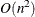memory except the conjugate gradient methods, which use only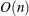of memory and are designed to optimize problems with many parameters. Since the techniques are iterative, they require the repeated computation of the following:

• the function value (optimization criterion)

• the gradient vector (first-order partial derivatives)

• for some techniques, the (approximate) Hessian matrix (second-order partial derivatives)

However, since each of the optimizers requires different derivatives, some computational efficiencies can be gained. The following table shows, for each optimization technique, which derivatives are required (FOD: first-order derivatives; SOD: second-order derivatives).

Algorithm

FOD

SOD

TRUREG

x

x

NEWRAP

x

x

NRRIDG

x

x

QUANEW

x

-

DBLDOG

x

-

CONGRA

x

-

NMSIMP

-

-

Each optimization method employs one or more convergence criteria that determine when it has converged. The various termination criteria are listed and described in the PROC NLMIXED Statement section. An algorithm is considered to have converged when any one of the convergence criteria is satisfied. For example, under the default settings, the QUANEW algorithm will converge if ABSGCONV< 1E–5, FCONV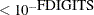, or GCONV
1E–8.

#### Choosing an Optimization Algorithm

The factors that go into choosing a particular optimization technique for a particular problem are complex and can involve trial and error.

For many optimization problems, computing the gradient takes more computer time than computing the function value, and computing the Hessian sometimes takes much more computer time and memory than computing the gradient, especially when there are many decision variables. Unfortunately, optimization techniques that do not use some kind of Hessian approximation usually require many more iterations than techniques that do use a Hessian matrix, and as a result the total run time of these techniques is often longer. Techniques that do not use the Hessian also tend to be less reliable. For example, they can more easily terminate at stationary points rather than at global optima.

A few general remarks about the various optimization techniques follow:

• The second-derivative methods TRUREG, NEWRAP, and NRRIDG are best for small problems where the Hessian matrix is not expensive to compute. Sometimes the NRRIDG algorithm can be faster than the TRUREG algorithm, but TRUREG can be more stable. The NRRIDG algorithm requires only one matrix with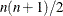double words; TRUREG and NEWRAP require two such matrices.

• The first-derivative methods QUANEW and DBLDOG are best for medium-sized problems where the objective function and the gradient are much faster to evaluate than the Hessian. The QUANEW and DBLDOG algorithms, in general, require more iterations than TRUREG, NRRIDG, and NEWRAP, but each iteration can be much faster. The QUANEW and DBLDOG algorithms require only the gradient to update an approximate Hessian, and they require slightly less memory than TRUREG or NEWRAP (essentially one matrix withdouble words). QUANEW is the default optimization method.

• The first-derivative method CONGRA is best for large problems where the objective function and the gradient can be computed much faster than the Hessian and where too much memory is required to store the (approximate) Hessian. The CONGRA algorithm, in general, requires more iterations than QUANEW or DBLDOG, but each iteration can be much faster. Since CONGRA requires only a factor of n double-word memory, many large applications of PROC NLMIXED can be solved only by CONGRA.

• The no-derivative method NMSIMP is best for small problems where derivatives are not continuous or are very difficult to compute.

#### Algorithm Descriptions

Some details about the optimization techniques follow.

##### Trust Region Optimization (TRUREG)

The trust region method uses the gradient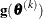and the Hessian matrix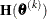; thus, it requires that the objective function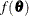have continuous first- and second-order derivatives inside the feasible region.

The trust region method iteratively optimizes a quadratic approximation to the nonlinear objective function within a hyperelliptic trust region with radius
that constrains the step size corresponding to the quality of the quadratic approximation. The trust region method is implemented using: Dennis, Gay, and Welsch (1981); Gay (1983); Moré and Sorensen (1983).

The trust region method performs well for small- to medium-sized problems, and it does not need many function, gradient, and Hessian calls. However, if the computation of the Hessian matrix is computationally expensive, one of the (dual) quasi-Newton or conjugate gradient algorithms might be more efficient.

##### Newton-Raphson Optimization with Line Search (NEWRAP)

The NEWRAP technique uses the gradientand the Hessian matrix; thus, it requires that the objective function have continuous first- and second-order derivatives inside the feasible region. If second-order derivatives are computed efficiently and precisely, the NEWRAP method can perform well for medium-sized to large problems, and it does not need many function, gradient, and Hessian calls.

This algorithm uses a pure Newton step when the Hessian is positive definite and when the Newton step reduces the value of the objective function successfully. Otherwise, a combination of ridging and line search is performed to compute successful steps. If the Hessian is not positive definite, a multiple of the identity matrix is added to the Hessian matrix to make it positive definite (Eskow and Schnabel, 1991).

In each iteration, a line search is performed along the search direction to find an approximate optimum of the objective function. The default line-search method uses quadratic interpolation and cubic extrapolation (LINESEARCH=2).

##### Newton-Raphson Ridge Optimization (NRRIDG)

The NRRIDG technique uses the gradientand the Hessian matrix; thus, it requires that the objective function have continuous first- and second-order derivatives inside the feasible region.

This algorithm uses a pure Newton step when the Hessian is positive definite and when the Newton step reduces the value of the objective function successfully. If at least one of these two conditions is not satisfied, a multiple of the identity matrix is added to the Hessian matrix.

The NRRIDG method performs well for small- to medium-sized problems, and it does not require many function, gradient, and Hessian calls. However, if the computation of the Hessian matrix is computationally expensive, one of the (dual) quasi-Newton or conjugate gradient algorithms might be more efficient.

Since the NRRIDG technique uses an orthogonal decomposition of the approximate Hessian, each iteration of NRRIDG can be slower than that of the NEWRAP technique, which works with Cholesky decomposition. Usually, however, NRRIDG requires fewer iterations than NEWRAP.

##### Quasi-Newton Optimization (QUANEW)

The (dual) quasi-Newton method uses the gradient, and it does not need to compute second-order derivatives since they are approximated. It works well for medium to moderately large optimization problems where the objective function and the gradient are much faster to compute than the Hessian; but, in general, it requires more iterations than the TRUREG, NEWRAP, and NRRIDG techniques, which compute second-order derivatives. QUANEW is the default optimization algorithm because it provides an appropriate balance between the speed and stability required for most nonlinear mixed model applications.

The QUANEW technique is one of the following, depending on the value of the UPDATE= option.

• the original quasi-Newton algorithm, which updates an approximation of the inverse Hessian

• the dual quasi-Newton algorithm, which updates the Cholesky factor of an approximate Hessian (default)

You can specify four update formulas with the UPDATE= option:

• DBFGS performs the dual Broyden, Fletcher, Goldfarb, and Shanno (BFGS) update of the Cholesky factor of the Hessian matrix. This is the default.

• DDFP performs the dual Davidon, Fletcher, and Powell (DFP) update of the Cholesky factor of the Hessian matrix.

• BFGS performs the original BFGS update of the inverse Hessian matrix.

• DFP performs the original DFP update of the inverse Hessian matrix.

In each iteration, a line search is performed along the search direction to find an approximate optimum. The default line-search method uses quadratic interpolation and cubic extrapolation to obtain a step sizesatisfying the Goldstein conditions. One of the Goldstein conditions can be violated if the feasible region defines an upper limit of the step size. Violating the left-side Goldstein condition can affect the positive definiteness of the quasi-Newton update. In that case, either the update is skipped or the iterations are restarted with an identity matrix, resulting in the steepest descent or ascent search direction. You can specify line-search algorithms other than the default with the LINESEARCH= option.

The QUANEW algorithm uses its own line-search technique. No options and parameters (except the INSTEP= option) controlling the line search in the other algorithms apply here. In several applications, large steps in the first iterations are troublesome. You can use the INSTEP= option to impose an upper bound for the step sizeduring the first five iterations. You can also use the INHESSIAN=r option to specify a different starting approximation for the Hessian. If you specify only the INHESSIAN option, the Cholesky factor of a (possibly ridged) finite difference approximation of the Hessian is used to initialize the quasi-Newton update process. The values of the LCSINGULAR=, LCEPSILON=, and LCDEACT= options, which control the processing of linear and boundary constraints, are valid only for the quadratic programming subroutine used in each iteration of the QUANEW algorithm.

##### Double-Dogleg Optimization (DBLDOG)

The double-dogleg optimization method combines the ideas of the quasi-Newton and trust region methods. In each iteration, the double-dogleg algorithm computes the step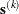as the linear combination of the steepest descent or ascent search direction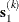and a quasi-Newton search direction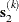: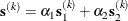The step is requested to remain within a prespecified trust region radius; see Fletcher (1987, p. 107). Thus, the DBLDOG subroutine uses the dual quasi-Newton update but does not perform a line search. You can specify two update formulas with the UPDATE= option:

• DBFGS performs the dual Broyden, Fletcher, Goldfarb, and Shanno update of the Cholesky factor of the Hessian matrix. This is the default.

• DDFP performs the dual Davidon, Fletcher, and Powell update of the Cholesky factor of the Hessian matrix.

The double-dogleg optimization technique works well for medium to moderately large optimization problems where the objective function and the gradient are much faster to compute than the Hessian. The implementation is based on Dennis and Mei (1979) and Gay (1983), but it is extended for dealing with boundary and linear constraints. The DBLDOG technique generally requires more iterations than the TRUREG, NEWRAP, and NRRIDG techniques, which require second-order derivatives; however, each of the DBLDOG iterations is computationally cheap. Furthermore, the DBLDOG technique requires only gradient calls for the update of the Cholesky factor of an approximate Hessian.

Second-order derivatives are not required by the CONGRA algorithm and are not even approximated. The CONGRA algorithm can be expensive in function and gradient calls, but it requires onlymemory for unconstrained optimization. In general, many iterations are required to obtain a precise solution, but each of the CONGRA iterations is computationally cheap. You can specify four different update formulas for generating the conjugate directions by using the UPDATE= option:

• PB performs the automatic restart update method of Powell (1977) and Beale (1972). This is the default.

• FR performs the Fletcher-Reeves update (Fletcher, 1987).

• PR performs the Polak-Ribiere update (Fletcher, 1987).

• CD performs a conjugate-descent update of Fletcher (1987).

The default, UPDATE=PB, behaved best in most test examples. You are advised to avoid the option UPDATE=CD, which behaved worst in most test examples.

The CONGRA subroutine should be used for optimization problems with large n. For the unconstrained or boundary constrained case, CONGRA requires onlybytes of working memory, whereas all other optimization methods require orderbytes of working memory. During n successive iterations, uninterrupted by restarts or changes in the working set, the conjugate gradient algorithm computes a cycle of n conjugate search directions. In each iteration, a line search is performed along the search direction to find an approximate optimum of the objective function. The default line-search method uses quadratic interpolation and cubic extrapolation to obtain a step sizesatisfying the Goldstein conditions. One of the Goldstein conditions can be violated if the feasible region defines an upper limit for the step size. Other line-search algorithms can be specified with the LINESEARCH= option.

The Nelder-Mead simplex method does not use any derivatives and does not assume that the objective function has continuous derivatives. The objective function itself needs to be continuous. This technique is quite expensive in the number of function calls, and it might be unable to generate precise results for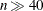.